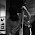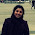Latest Articles

# To calculate 1/19 ,1/29,1/39 and so on

Info Post

The numbers like 19, 29, 59 are prime numbers and so cannot be factorised and division becomes more difficult and runs into many pages in the present conventional method and the chances of making mistakes are many.But in the Vedic way, the solution is obtained by applying the Sutra (theorem) Ekadhikena Purvena which when translated means "One more than the Previous".Lets see them in two different ways.You can apply whichever is easiest for you.

Way 1 : Using multiplication to calculate 1/19
To find the decimal expansion of 1/19, one more than the number before the 9 in the denominator is 2..Also number 19 is not divisible by 2 or 5(since decimal fractions can be found for prime numbers other than 2 or 5), the fractional result is a purely circulating decimal.

Note : One more than the one before here means one more than the number before the 9 in the denominator. One more than 1 is 2. So we repeatedly multiply by 2

Now starting with the last digit of the result, being the dividend-
1
Multiply this by "one more", that is, 2 (this is the "key" digit from 'Ekadhikina')
2 1
Keep mutiplying by 2 to the above number we get

421
then 8421
then 168421 ....carry over '1' keeping the unit digits ,so

68421 with carry over '1'...again multiply by 2

=  ( 12 +1)68421
=  1368421 ...carry over '1' again and multiply with 2

= 7368421 → 47368421 → 947368421.... until we get a nine digit number

To get a total of 18 digits for the answer,we have to complement the lower half with '9'

947368421
052631578 ...adding both above and below gives the number 999999999

Way 2 : Using division to calculate 1/19

We start again with 1 (dividend of "1/x9"), dividing by 2 (" x + 1 "). The previous digit of 9 is 1 ..so we have divide by one more than 1 ,that is ,we divide  by 2,

we get answer as 0 with remainder 1,we make it as  remainder,quotient thats is  10

Now divide 10  by 2  we get remainder and quotient as 0 and 5
.05 ...taking the quotients only
Now divided .05 by 2  we get remainder and quotient as  1 and 2
.05  2
Now repeating the same ,we get remainder 0 and quotient   is 6
.0526
and so on.

NOTE:
Similarly, if you want the decimal expansion of 1/29, one more than the number before the 9 in the denominator is 3

Multiply this by "one more", that is, 3 (this is the "key" digit from 'Ekadhikina')
3 1
Keep mutiplying by 3 from the left hand side till we get a fourteen digit number (since half of 28 is 14...by using one less than the previous i.e:29 -1 =28)

= (carry 1)137931→ (carry 2) 1724137931→2896551724137931 till we get a fourteen DIGIT number
i.e : 96551724137931

To get a total of 28 digits for the answer(one less than the previous i.e:29 -1 =28),we have to complement the lower half with '9'
96551724137931
03448275862068 ...adding both above and below gives the number 99999999999999
Therefore, 1/29 = 0.0344827586206896551724137

We can use this method for finding (1/39),(1/49)......(119), etc  where the factors are 4,5 ....12 etc keeping in mind that it follows the pattern ( 1/ a9) , where a is any value of n and 9 is the last digit of the number .

Share:

1.2.function with 29, tried with 39 and fail, can you help me?

1.Answered below. Thanks for visiting :)

3.Tried with 29 and function flawlesly, then tried 39 and failed (de 20th digit get negative?), can you help me. Will be greatly apreciated

1.For 39, our multiplier is 4 which is got by 3+1 =4 . The numerator remains the same .
Now solving (1/39 ) using Vedic maths,

1
41 [ 4x1]
641 [ 4x4 =16, we keep 6 and carry over "1" ]
5641 [ 4x6 =24 +carried "1" from above makes 25 , keep 5 and carry "2" now]
25641 [ 4x5 =20+ carried "2" from above makes 22 , keep 2 and carry "2" again]
025641 [ 4x2=8+ carried "2" from above makes 10, keep 0 and carry "1"]
1025641 [ 4x0=0 +carried "1" makes 1]
Here, we stop, since we get remainder zero and any continuation would just brings repetition.